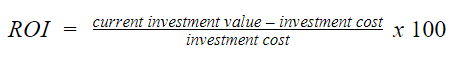# What is the ROI Formula? How to Calculate ROIReturn on investment (ROI) is a measure of the profitability of a particular investment.

## How is ROI calculated?

You can calculate ROI by dividing the return of an investment by the cost. It can be expressed as a ratio or as a percentage. The ROI formula is:For example, an investor buys one share of Jet Airlines Corp. for \$500. Three years later, the investor sells the share for \$750. The current investment value (\$750) minus the investment cost (\$500) equals \$250. \$250 divided by the investment cost (\$500) equals \$0.50. Therefore, the ROI on the Jet Airlines investment is 50%.

## What does ROI fail to include?

The main pitfall of the ROI calculation is that it fails to include the amount of time. To demonstrate this, let’s look at another example.

An investor purchases one share of Sonic Sneakers Inc. for \$10 and sells it ten years later for \$15. The current investment value (\$15) minus the investment cost (\$10) equals \$5. \$5 divided by the investment cost (\$10) equals \$0.50. Therefore, the ROI on the Sonic Sneakers investment is also 50%.

The ROI for both the Jet Airlines and Sonic Sneakers investments is 50%. One may look at this and assume there is no difference between the two. However, this is a mistake because the two investments were calculated in different time ranges.

The 50% return on the Jet Airlines investment was achieved in just three years. It took an entire decade to reach the same ROI on the Sonic Sneakers investment. Although both have the same ROI, the Jet Airlines investment is clearly the better option.

To include the consideration of time, calculate ROI on an annualized basis. The formula for annualized ROI is:## What is an acceptable ROI?

There is no magic percentage point that makes an investment’s ROI acceptable. ROI averages vary based on factors like industry, type of investment, and time range. An investment with a negative ROI should be avoided.

Risk tolerance and time are factors an investor should consider when determining whether an investment’s ROI is satisfactory. Investors with a low risk tolerance are typically content with a lower ROI. Higher risk investors typically want to see a higher ROI for taking on more risk. Investors often desire a higher ROI for an investment that takes longer to pay off.

## What is ROI used for?

ROI is not only for measuring stock market investments. It’s a flexible formula that has several other purposes. You also can use the ROI formula to measure the return on investments in real estate, cryptocurrency, and startups.

It’s a critical measurement business owners use to evaluate investments. For example, High Performance Energy Co. hires a new sales representative at a salary of \$100,000 per year. This sales representative is directly responsible for \$300,000 in sales during the first year.

This sales representative brought in \$300,000 per year while only costing the company \$100,000. The ROI for this “investment” is 200%. While this is a simplified example, it shows one way that companies measure their investments. Businesses will also use ROI to measure investments on things like equipment, marketing campaigns, and software platforms.

ROI is a simple formula that measures the profitability of an investment. Calculate ROI by subtracting an investment’s cost from its current value, then dividing it by the investment’s cost.

ROI does not consider an investment’s time range, but the annualized ROI formula is an alternative calculation that does. A good ROI varies depending on factors like risk tolerance, investment type, and amount of time.

Return on investment is a basic yet critical formula used by financial investors, business owners, and angel investors

You may also like# www.cbinsights.com